login  home  contents  what's new  discussion  bug reports help  links  subscribe  changes  refresh  edit

fricas
--- Functions to facilitate Transforming Polynomial Generating Functions into
---- Coefficient arrays.  With some examples
--- These are coefficient arrays (A) and can be rendered into a polynomial list
--- by A*[1,x,x^2,...]^T
--- EGF Validation can always done for f(x,t)
--- By doing a Taylor series expansion indexed by t
--- OGF/Power Series is similiar except the factorial n! in t^n/n!
--- has to be applied to the polynomial.
--- Little error checking.  Most Functions expect UniPotent of Nilpotent
--- Arrays.
---
--- The base ideas come from generating functions like
--- f(t)*exp(x*g(t))
--- From Roman's "Umbral Calculus" and
--- The Matrices of Pascal and Other Greats
--- Lidia Aceto & Donato Trigiante
--- A, somewhat tedious, introduction to the mathematics is at:
---https://www.dropbox.com/s/tb9j7z0nhrdq92k/H-generating-9.pdf?dl=0
--- https://www.dropbox.com/sh/i2f7lehirme848p/AAA3jUgIkLNshPK88HuOR4MJa?dl=0
--- H-generating-9.pdf
---
---
---)Clear all
--- Complex here to accomidate cos(),sin() sequences
FPFI ==> Fraction(Polynomial(Fraction(Complex(Integer))))
Type: Void
fricas
---
---
--- Lower strict Jordan
--- When used on the left of variables x it divides by x
--- with 1/x =0
--- When used on the right of the coefficient matrix it shifts
--- the columns to the left by one.
--- Really a horizontal shift of the coefficient array and
--- Should be applied A*SJ_lower*[x...]^T
---
SJ_lower(dim) ==
J : Matrix(Polynomial(Fraction(Integer))) := new(dim,dim,0)
for i in 1..(dim-1) repeat J(i+1,i):=1
J
Type: Void
fricas
---
---
--- Upper strict Jordan
--- When used on the left of variables x it multiplies by x
--- With last entry 0
--- When used on the right of coefficient matrix it shifts
--- the columns to the right by one.
---
SJ_upper(dim) ==
J : Matrix(FPFI) := new(dim,dim,0)
for i in 1..(dim-1) repeat J(i,i+1):=1
J
Type: Void
fricas
---
---
--- Matrix exponential limited for now
--- Returns exp(Mat)
---
Exp_Matrix(Mat) ==
n:=nrows(Mat)
Mat_ret :Matrix(FPFI) := Mat^0
for i in 1..(n) repeat Mat_ret := Mat_ret +(Mat^i)/factorial(i)
Mat_ret
Type: Void
fricas
---
---
--- Id matrix
--- Exp_Matrix defined below
---
Id_M(dim) ==
exp_tmp: Matrix(Integer) := new(dim,dim,0)
exp :=Exp_Matrix(exp_tmp)
exp
Type: Void
fricas
---
---
--- H and Pascals matrix
---
Gen_H(dim) ==
H : Matrix(FPFI) := new(dim,dim,0)
for i in 1..(dim-1) repeat H(i+1,i):=i
H
Type: Void
fricas
---
---
---Generate Pascal Matrices with a scalar (x) coefficient
--- exp(x*H)
---
Gen_Pascal(x,dim) ==
H : Matrix(FPFI):=x*Gen_H(dim)
Exp_Matrix(H)
Type: Void
fricas
---
---
---H Left handed Psuedo inverse H_PIl
--- H_PIl*H ~ I
--- inverts H except for the last row.
---
Gen_H_PIl(dim) ==
H_PIl : Matrix(FPFI) := new(dim,dim,0)
for i in 1..(dim-1) repeat H_PIl(i,i+1):=1/i
H_PIl
Type: Void
fricas
---
---
--- Calculate Roman's integral equation
--- (exp(x*H)-1)/H
--- Which must be done in proper order
--- Note that in order for the last row
--- to be correct the expansion must be done
--- to dim+1 and then is trimmed to dim.
---
Gen_RI(x,dim) ==
RI := Gen_Pascal(x,dim+1)
RI := RI-RI^0
RI := Gen_H_PIl(dim+1)*RI
RI := subMatrix(RI,1,dim,1,dim)
RI
Type: Void
fricas
---
---
--- Log of particular matrix
--- Requires A - I be nilpotent and square;
--- i.e. A is Unipotent/Lower unittriangular
---
Ln_Uni_Matrix(A) ==
n := nrows(A)
m := ncols(A)
if n=m then
A1 := A-A^0
A_ret : Matrix(FPFI) := new(n,n,0)
if (A1^n = A_ret) then
for i in 1..n repeat A_ret:=A_ret+(-A1)^i/i
else
else
output("Ln_nil_matrix entered with non-square matrix")
output(A)
-A_ret
Type: Void
fricas
---
--- (A^B) A a matrix, B either integer or matrix
--- A must be Unipotent
---
Exp_Matrix_AB(A,B) ==
Exp_Matrix(Ln_Uni_Matrix(A)*B)
Type: Void
fricas
---
--- Find p in P^-1* A* P = J
--- J is really a shift
--- A must be Stricly Lower Triangular
---
ST_J(A) ==
n:=nrows(A)
m:=ncols(A)
P : Matrix(FPFI) := new(n,n,0)
if (n=m) and (A^n = P) then
P(1,1):=1
for i in 1..(n-1) repeat P(1..n,i+1):= A*P(1..n,i)
else
output("Input to ST_H not strictly triangular")
P
Type: Void
fricas
---
--- Same as ST_H except the equation is P^-1*A *P=H
---
ST_H(A) ==
n:=nrows(A)
m:=ncols(A)
P : Matrix(      FPFI) := new(n,n,0)
if (n=m) and (A^n = P) then
P(1,1):=1
for i in 1..(n-1) repeat P(1..n,i+1):= A*P(1..n,i)/(i)
else
output("Input to ST_H not strictly triangular")
P
Type: Void
fricas
---
---
--- Start of Examples
---
dim:=5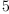(1)
Type: PositiveInteger?
fricas
H:=Gen_H(dim)
fricas
Compiling function Gen_H with type PositiveInteger -> Matrix(
Fraction(Polynomial(Fraction(Complex(Integer)))))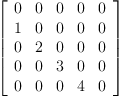(2)
Type: Matrix(Fraction(Polynomial(Fraction(Complex(Integer)))))
fricas
GP :=Gen_Pascal(1,dim)
fricas
Compiling function Exp_Matrix with type Matrix(Fraction(Polynomial(
Fraction(Complex(Integer))))) -> Matrix(Fraction(Polynomial(
Fraction(Complex(Integer)))))
fricas
Compiling function Gen_Pascal with type (PositiveInteger,
PositiveInteger) -> Matrix(Fraction(Polynomial(Fraction(Complex(
Integer)))))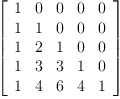(3)
Type: Matrix(Fraction(Polynomial(Fraction(Complex(Integer)))))
fricas
Id := GP^0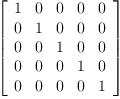(4)
Type: Matrix(Fraction(Polynomial(Fraction(Complex(Integer)))))
fricas
--- Enough is enough in taylor stream
fricas
)set streams calculate 4
t := series 't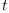(5)
Type: UnivariatePuiseuxSeries?(Expression(Integer),t,0)
fricas
--- To change a coefficient array to the corresponding polynomial array
XC := matrix[,[x],[x^2],[x^3],[x^4]]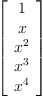(6)
Type: Matrix(Polynomial(Integer))
fricas
---
---
--- Appell Sequences
--- f(t)*exp(x*t)
---
--- Euler Polynmials from WikiPedia
--- 2/(e^t +1) exp(x*t)
---
Euler_A:=2*((GP+1)^-1)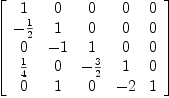(7)
Type: SquareMatrix?(5,Fraction(Integer))
fricas
--- Test
Euler_A*XC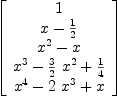(8)
Type: Matrix(Polynomial(Fraction(Integer)))
fricas
---
--- Bernoulli Polynomials from WikiPedia
--- (t/(e^t -1))* exp(x*t)
--- We recognize the this is Roman's integral formula
--- and we have 0/0 for t/H=0 and requires special
--- handling as noted above.
---
Bern_Wiki_A := Gen_RI(1,dim)^-1
fricas
Compiling function Gen_H_PIl with type PositiveInteger -> Matrix(
Fraction(Polynomial(Fraction(Complex(Integer)))))
fricas
Compiling function Gen_RI with type (PositiveInteger,
PositiveInteger) -> Matrix(Fraction(Polynomial(Fraction(Complex(
Integer)))))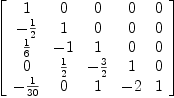(9)
Type: Matrix(Fraction(Polynomial(Fraction(Complex(Integer)))))
fricas
---
--- Bernoulli of order a from Roman
------ (t/(e^t -1))^a * exp(x*t)
---- This is the same as above exponenitated
---
Bern_Roman_A := Exp_Matrix_AB(Bern_Wiki_A,a)
fricas
Compiling function Ln_Uni_Matrix with type Matrix(Fraction(
Polynomial(Fraction(Complex(Integer))))) -> Matrix(Fraction(
Polynomial(Fraction(Complex(Integer)))))
fricas
Compiling function Exp_Matrix_AB with type (Matrix(Fraction(
Polynomial(Fraction(Complex(Integer))))), Variable(a)) -> Matrix(
Fraction(Polynomial(Fraction(Complex(Integer)))))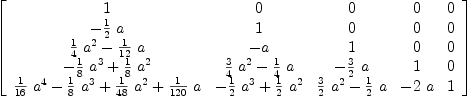(10)
Type: Matrix(Fraction(Polynomial(Fraction(Complex(Integer)))))
fricas
---
----
---- From
----Some Identities for Euler and Bernoulli Polynomials
----and Their Zeros ---equation 4.
--- by
----Taekyun Kim and Cheon Seoung Ryoo
----Frobenius–Euler polynomials of order r
---------- (1-u)/(e^t -u))^r * exp(x*t)
----
Frob_Euler_term:=((1-u)*Id_M(dim))*((Gen_Pascal(1,dim)-u*Id_M(dim))^-1);
fricas
Compiling function Exp_Matrix with type Matrix(Integer) -> Matrix(
Fraction(Polynomial(Fraction(Complex(Integer)))))
fricas
Compiling function Id_M with type PositiveInteger -> Matrix(Fraction
(Polynomial(Fraction(Complex(Integer)))))
Type: Matrix(Fraction(Polynomial(Fraction(Complex(Integer)))))
fricas
Frob_Euler:=Exp_Matrix_AB(Frob_Euler_term,r)
fricas
Compiling function Exp_Matrix_AB with type (Matrix(Fraction(
Polynomial(Fraction(Complex(Integer))))), Variable(r)) -> Matrix(
Fraction(Polynomial(Fraction(Complex(Integer)))))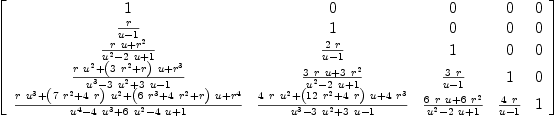(11)
Type: Matrix(Fraction(Polynomial(Fraction(Complex(Integer)))))
fricas
---
---
--- Hermite Polynomials wiki version
--- Probabilistic version
---exp(-(t^2)/2) *exp(x*t)
---
Herm_Wiki_A := Exp_Matrix(-H^2/2)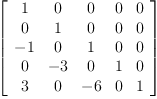(12)
Type: Matrix(Fraction(Polynomial(Fraction(Complex(Integer)))))
fricas
--- Roman's version
Herm_Roman_A := Exp_Matrix(-v*H^2/2)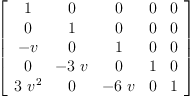(13)
Type: Matrix(Fraction(Polynomial(Fraction(Complex(Integer)))))
fricas
--- Hermite/Gould Hopper of order 3
--- exp(y*t^3)*exp(x*t)
HGH_A := Exp_Matrix(y*H^3)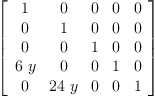(14)
Type: Matrix(Fraction(Polynomial(Fraction(Complex(Integer)))))
fricas
---
---
--- Associated Sequences requires Jordan transform
--- exp(x*f(t)) -> SJ_H(f(H))
---
--- Lower Factorial Exponential
--- Roman PP 57
--- exp(x*Ln(t+1))
---
Lower_Fact_Exp_A := ST_H(Ln_Uni_Matrix(H+1))
fricas
Compiling function Ln_Uni_Matrix with type SquareMatrix(5,Integer)
-> Matrix(Fraction(Polynomial(Fraction(Complex(Integer)))))
fricas
Compiling function ST_H with type Matrix(Fraction(Polynomial(
Fraction(Complex(Integer))))) -> Matrix(Fraction(Polynomial(
Fraction(Complex(Integer)))))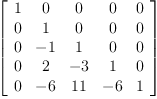(15)
Type: Matrix(Fraction(Polynomial(Fraction(Complex(Integer)))))
fricas
--- Just to verify
x:='x;
Type: Variable(x)
fricas
1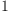(16)
Type: PositiveInteger?
fricas
x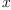(17)
Type: Variable(x)
fricas
x*(x-1)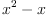(18)
Type: Polynomial(Integer)
fricas
x*(x-1)*(x-2)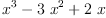(19)
Type: Polynomial(Integer)
fricas
x*(x-1)*(x-2)*(x-3)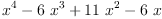(20)
Type: Polynomial(Integer)
fricas
---
---  Exponential Polynomials
--- Roman PP 64
--- Also http://mathworld.wolfram.com/BellPolynomial.html
--- exp(x*(exp(t)-1))
---
Exp_A := ST_H(GP-1)
fricas
Compiling function ST_H with type SquareMatrix(5,Integer) -> Matrix(
Fraction(Polynomial(Fraction(Complex(Integer)))))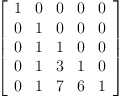(21)
Type: Matrix(Fraction(Polynomial(Fraction(Complex(Integer)))))
fricas
---
---
-(22)
Type: Variable(-)
fricas
--- Scheffer Sequences require Jordan form
---
--- g(t)*exp(x*f(t)) ->g(H)*ST_H(f(H))
---
--- Laguerre Polynomials Roman PP 110
--- (1-t)^(-a-1)*exp(x*(t/(t-1)))
Lag_A  := Exp_Matrix_AB(1-H,(-a-1))*ST_H(H*(H-1)^-1)
fricas
Compiling function Exp_Matrix_AB with type (SquareMatrix(5,Integer)
, Polynomial(Integer)) -> Matrix(Fraction(Polynomial(Fraction(
Complex(Integer)))))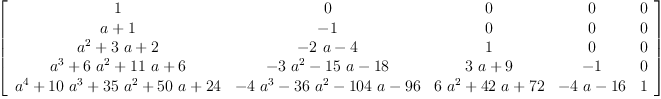(23)
Type: Matrix(Fraction(Polynomial(Fraction(Complex(Integer)))))
fricas
--- Checking
Lag_S:=(1-t)^(-a-1)*exp(x*(t/(t-1)));
Type: UnivariatePuiseuxSeries?(Expression(Integer),t,0)
fricas
Lag_S_C3:=6*coefficient(Lag_S,3)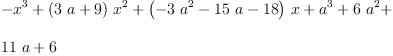(24)
Type: Expression(Integer)
fricas
Lag_P:=Lag_A*XC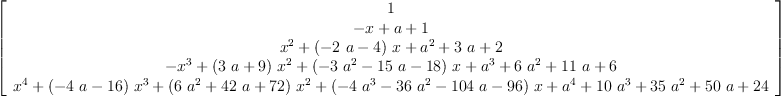(25)
Type: Matrix(Fraction(Polynomial(Fraction(Complex(Integer)))))
fricas
Lag_P(4,1)-Lag_S_C3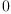(26)
Type: Expression(Complex(Integer))
fricas
--- Note the "series" give "Power Series" to get the
--- Taylor series one has to multiply by factorial(n)
---
--- You might want to comment this out; it has a lot of terms  at the end.
--- Meixner Polynomials
--- Acuctually this is interesting since it is
--- Listed as Scheffer (which is Exponential)
--- but I think Ordinary OGF is a better choice
--- Anyhow here is a slight reformat from Roman
t := series 't(27)
Type: UnivariatePuiseuxSeries?(Expression(Integer),t,0)
fricas
Meix_Series_R :=((1-t*d)*((1-t)^-1))^x*(1-t)^(-b)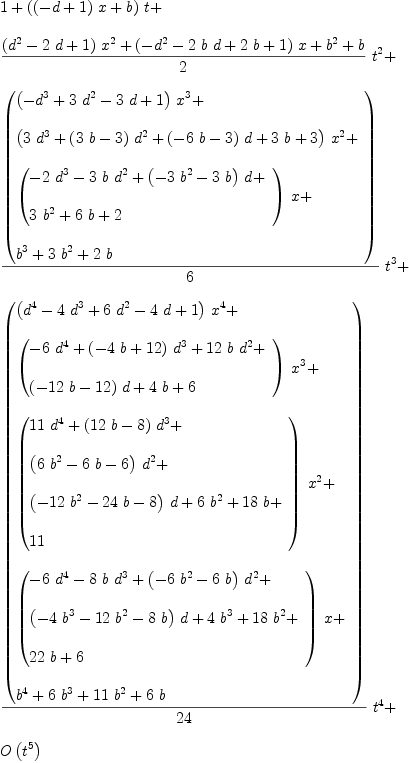(28)
Type: UnivariatePuiseuxSeries?(Expression(Integer),t,0)
fricas
--- and the Sheffer form
--- We need the Jordan form of Ln_Uni_Matrix((1-H*d)*(1-H)^-1)
Meix_A_fJ := ST_H(Ln_Uni_Matrix((1-H*d)*(1-H)^-1))
fricas
Compiling function Ln_Uni_Matrix with type SquareMatrix(5,Polynomial
(Fraction(Complex(Integer)))) -> Matrix(Fraction(Polynomial(
Fraction(Complex(Integer)))))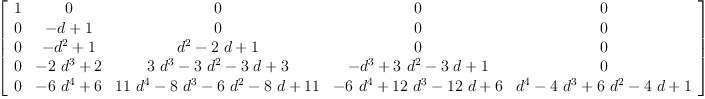(29)
Type: Matrix(Fraction(Polynomial(Fraction(Complex(Integer)))))
fricas
Meix_A := Exp_Matrix_AB(1-H,(-b))*Meix_A_fJ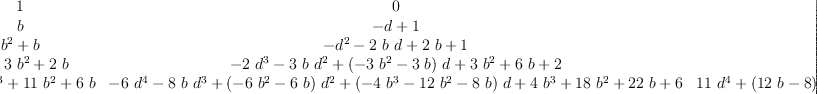(30)
Type: Matrix(Fraction(Polynomial(Fraction(Complex(Integer)))))
fricas
---
--- Let's check
Meix_C3:=6*coefficient(Meix_Series_R,3)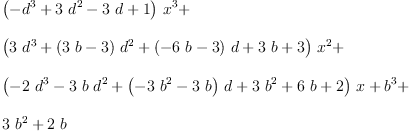(31)
Type: Expression(Integer)
fricas
Meix_P:=Meix_A*XC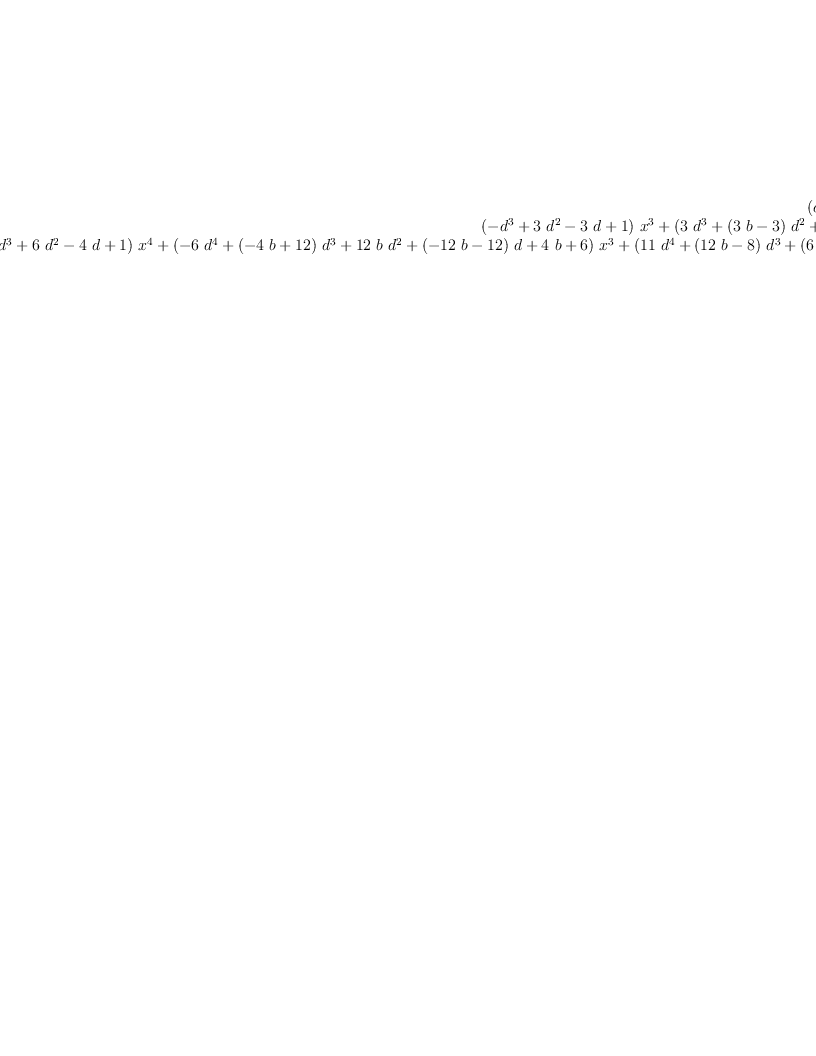(32)
Type: Matrix(Fraction(Polynomial(Fraction(Complex(Integer)))))
fricas
Meix_P(4,1)-Meix_C3(33)
Type: Expression(Complex(Integer))

 Subject:   Be Bold !! ( 14 subscribers )